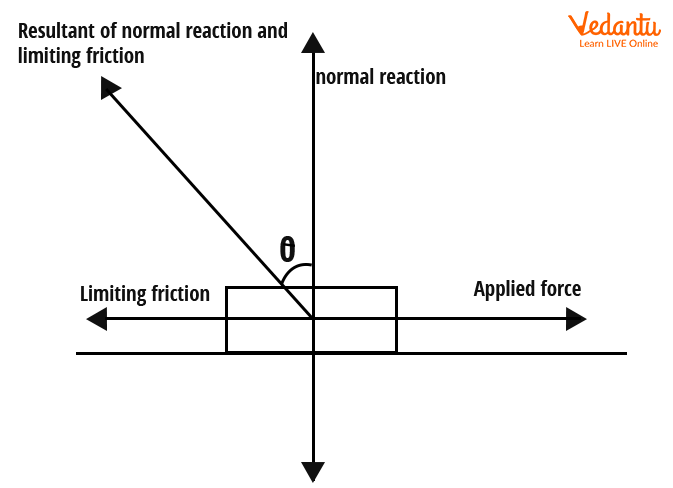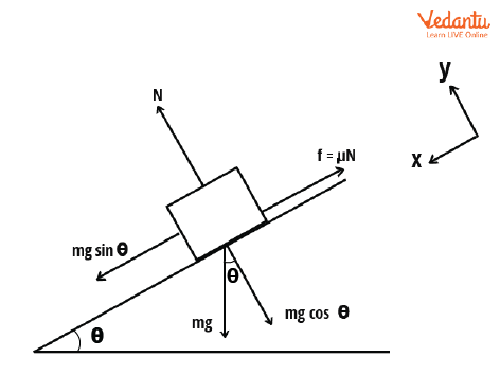Courses
Courses for Kids
Free study material
Free LIVE classes
More

# Angle of Repose - Important Topic for JEELIVE
Join Vedantu’s FREE Mastercalss

## What is Friction?

Before moving on to the angle of repose, it is important to understand the concept of friction and coefficient of friction because the angle of repose is calculated using the coefficient of friction. Friction is a contact force that opposes the relative motion between any two surfaces. When the surface of one body slides over the surface of another body, both these bodies experience a contact force that is opposite to the relative motion between the surfaces. This opposing force is the frictional force. The reason for the friction is the intermolecular interaction that arises due to the elastic properties of matter. The frictional force acts tangentially to the interface of the surfaces of two bodies.

Earlier it was thought that the interlocking of irregularities in the surfaces was the cause of friction. But now experiments have relieved that the actual contact area between the surfaces is much less than the overall area in contact. It is now thought that at the points of contact, very small and cold-welded joints are formed due to the strong adhesive forces between the molecules of the surfaces that are in close contact.

Friction depends on the nature of two surfaces in contact, the normal force with the surfaces that are in contact, and the actual area of contact between the surfaces.

## Coefficient of Friction

When two surfaces are in contact, there is a force of friction between them. The coefficient of friction indicates the amount of interaction between the two surfaces in contact. It can be said that the coefficient of friction indicates the roughness of the surfaces. Any object that is subjected to a normal force experiences a force of friction. This frictional force can be expressed mathematically by the law of limiting friction. The law states that the force of friction is directly proportional to the normal force that is impressed on the object. Now if F is the force of frictional and N is the normal force on the object, then the relationship can be written mathematically as,

$F\propto N$

A constant of proportionality can be introduced in the above expression. The equation would then be,

$F=\mu N$

Here in the above equation $\mu$ is the coefficient of limiting friction between the two surfaces that are kept in contact.

The value of $\mu$ can be written as,

$\mu=\dfrac{F}{N}$

So the coefficient of limiting friction can be defined as the ratio of the force of limiting friction and the normal reaction or force between the surfaces in contact. The value of the coefficient of limiting friction μ depends on:

• The nature of the surfaces in contact. The coefficient depends on whether the surfaces are dry, wet, rough, smooth, polished, or not polished. All these properties have an impact on the value of $\mu$.

• The materials that are in contact. If the materials are smooth such as glass, then the coefficient of friction is less and if the materials are rough such as wood, the value of $\mu$ is more.

## Angle of Friction

The angle of friction is defined as the angle that is made between the resultant of frictional force and the normal reaction and the normal force. The angle of friction is shown in the diagram below.Angle of friction

When any force is applied to the body, the angle of friction can be seen in the diagram above. Now if the coefficient of friction between the surfaces is $\mu$, we know that the coefficient of friction can be defined as the ratio of the frictional force to the normal force. This can be written as,

$\mu=\dfrac{F_f}{N}$

Here Ff is the force of friction and N is the normal reaction.

We can now determine the value of $\tan{\theta}$ as the ratio of force in the horizontal direction to the ratio of force in the vertical direction using the block's schematic diagram. We can write the friction force Ff in the horizontal direction and the normal force N in the vertical direction because the friction force Ff acts in the horizontal direction and the normal force N acts in the vertical direction. Mathematically we’ll have,

$\tan{\theta}=\dfrac{F_f}{N}$

Since $\dfrac{F_f}{N}$ is equal to $\mu$, we can write

$\tan{\theta}=\mu$

So the angle of friction can be written as,

$\theta=\tan ^{-1}\mu$

## Angle of Repose

The angle of repose is defined as the angle formed by an inclined plane with the horizontal when a body is about to slide down it. Consider a body of mass m that is positioned on a rough inclined plane that has an inclination angle $\theta$ and a coefficient of friction $\mu$. This body is shown in the diagram below.Angle of repose calculation

We now apply the equilibrium of forces that are along the y-direction. This gives,

$N-mg\cos{\theta}=0$

$N=mg\cos{\theta}$

Here g is the acceleration due to gravity and $\theta$ is the angle of inclination.

Similarly applying the equilibrium of forces along the x-direction gives,

\begin{align} &m g \sin \theta-\mu N=0 \\ &m g \sin \theta=\mu N \end{align}

Now we have $mg \cos \theta=N$, and substituting this value of N in the above equation gives,

$mg\sin{\theta}=\mu mg\cos{\theta}$

Solving this equation further gives,

\begin{align} &\mu=\dfrac{\sin \theta}{\cos \theta} \\ \\ &\mu=\tan \theta \end{align}

This means that the tangent of the angle of repose is equal to the coefficient of friction between the block and the plane.

One thing to be noted is that we define the angle of friction and angle of repose differently, but they both have the same value. Someone could ask which property is defined by the angle of repose and the answer is that the tangent of the angle of repose defines the coefficient of friction between the surfaces.

## Conclusion

Friction is defined as the contact force which opposes the relative motion between any two surfaces in contact. The value of friction depends on the type of surfaces in contact and their coefficient of friction and also the normal reaction between the surfaces. The angle of friction is the angle between the resultant of the frictional force and normal reaction and the normal force. The angle of repose is defined as the angle of inclination with the horizontal when the body is on an inclined plane. The angle of friction and angle of repose both have the same value as the tangent of these angles is equal to the coefficient of friction.

Last updated date: 26th Sep 2023
Total views: 2.1k
Views today: 0.02k

## FAQs on Angle of Repose - Important Topic for JEE

1. Why is the angle of repose important?

In the design of systems for the processing, storage, and transporting of particulate materials, the angle of repose is extremely significant. When the grains are smooth, the coefficient of friction is low, and hence the angle of repose is low. However, if the grains are sticky, the high coefficient of friction causes the angle of repose to increase. If the angle of repose is known, it can also be used to calculate the coefficient of friction between the surfaces that are kept in contact.

2. What is the significance of the angle of friction?

The angle of friction can be defined in another way as the minimum angle of incline that anybody can rest on a surface without sliding. Because any surface's coefficient of friction can only be between 0 and 1, the angle of friction can only be between 0 and 90 degrees. If the angle between the block and the surface is smaller than the angle of friction, the block will begin to slide on the surface.